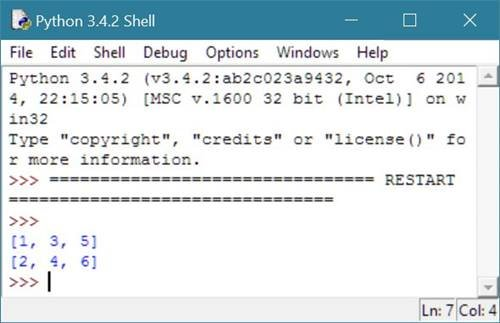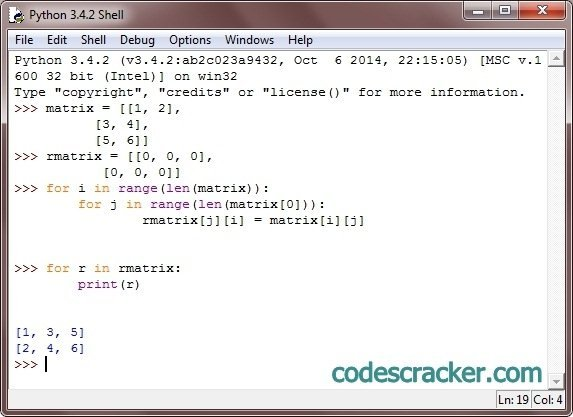# Python Program to Find Transpose of Matrix

## Find Transpose of Matrix in Python

To find transpose of a matrix in python, just choose a matrix which is going to transpose, and choose another matrix having column one greater than the previous matrix and row one less than the matrix. Initially second matrix will be empty matrix. Now find the transpose of matrix and print the transpose result as output.

## Python Programming Code to Find Transpose of Matrix

Following python program shows how to find and print the transpose of a matrix:

```# Python Program - Find Transpose of a Matrix

matrix = [[1, 2],
[3, 4],
[5, 6]]
rmatrix = [[0, 0, 0],
[0, 0, 0]]
for i in range(len(matrix)):
for j in range(len(matrix)):
rmatrix[j][i] = matrix[i][j]
for r in rmatrix:
print(r)
```

Transpose of a matrix can be calculated as exchanging row by column and column by row's elements, for example in above program the matrix contains all its elements in following ways:

• matrix = 1
• matrix = 2
• matrix = 3
• matrix = 4
• matrix = 5
• matrix = 6

Now the transpose of the above matrix can be done in this way:

• rmatrix = matrix = 1
• rmatrix = matrix = 3
• rmatrix = matrix = 5
• rmatrix = matrix = 2
• rmatrix = matrix = 4
• rmatrix = matrix = 6

Here is the sample run of the above python program:Below is same program on python shell:### Same Program in Other Languages

You may also like to learn or practice the same program in other popular programming languages:

Tools
Calculator